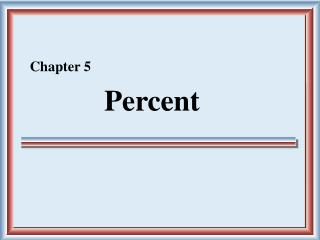DownloadDownload PresentationPercent

# Percent

Télécharger la présentation## Percent

- - - - - - - - - - - - - - - - - - - - - - - - - - - E N D - - - - - - - - - - - - - - - - - - - - - - - - - - -
##### Presentation Transcript

1. Chapter 5 Percent

2. § 5.1 Understanding Percent

3. Denominators of 100 The word percent means “per hundred.” A percent is another way to describe a part of a whole. 7 out of 100 of the rectangles are shaded. 7 percent of the whole is shaded.

4. Example: Write as a percent. Example: Write as a percent. Denominators of 100

5. Writing Percents as Decimals Percents can be written as fractions and decimals. Changing a Percent to a Decimal • Drop the % symbol. • Move the decimal point two places to the left.

6. Writing Percents as Decimals Example: Write 19% as a decimal. 19% = 19. . = 0.19 Example: Write 2.67% as a decimal. An extra zero is added to the left of the 2. 2.67% = 2.67 . = 0.0267 Example: Write 0.23% as a decimal. Two extra zeroes are added to the left of the 2. 0.23% = 0.23 . = 0.0023

7. Writing Decimals as Percents Changing a Decimal to a Percent • Move the decimal point two places to the right. • Add the % symbol at the end of the number. Example: Write 0.25 as a percent. . 0.25 = 0.25 = 25% Example: Write 3.95 as a percent. . 3.95 = 3.95 = 395%